Example of working a quiz - D1L1 Batch Quiz

These are screen shots on a Mac, the appearance on a Windows PC is very similar.

After starting the Reactor Lab application, you will go to the Reactor Lab Directory. When you first use the Lab, click on the info button at the top of the window to get advice on using the lab. Then click on "L1 Nth Order Reactions" in the lab listing: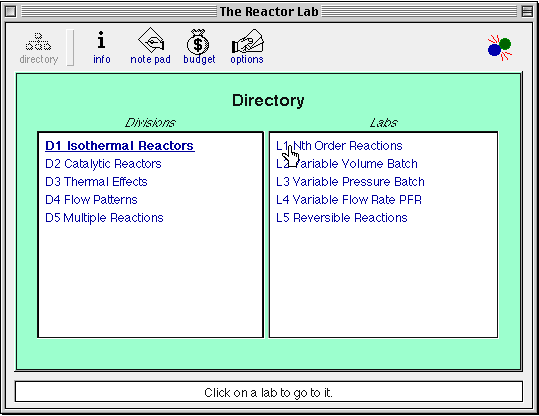On the first screen of L1 (not shown here), click on the "batch" reactor button. This will take you to the Batch reactor lab. In the regular "lab" you know all the input values. Slide the cursor around the window and read what different objects do in the field at the bottom of the window. Click on the "info" button to get information specific to this lab (e.g., an equivalent "space time" for a batch reactor with zero changeover time is given for comparison of batch results to PFR and CSTR results). Make some runs here to test your understanding and test the equations you plan to use. When you are ready to work the lab quiz, click on the "quiz" button at the top to go to the quiz.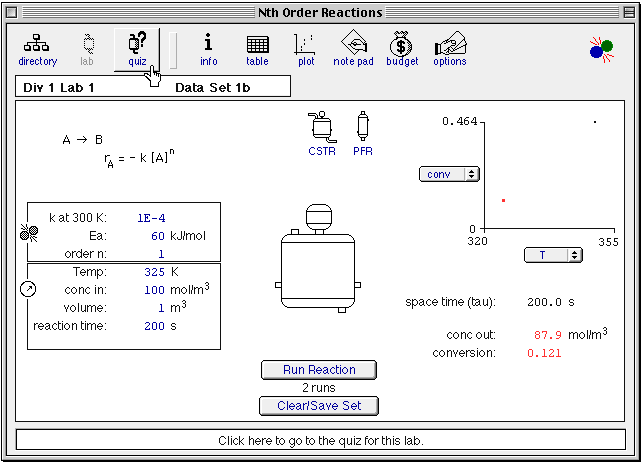Here is the Batch reactor quiz:In this example, we want to keep things as simple as possible, so we will specify the reaction order is 1 rather than leaving the order as an unknown. Click the "Quiz Options" button and, if n = 1 is not default in your version, click the "order n" input value to specify that you want the reaction order to be 1.You will probably have to vary conditions in your reactor (T, reaction time) in order to get the conversion within the range 10-90% so that you can get a good estimate of the reaction rate. Note that there is random error present in the output values. This random error can result in small negative values of the reported conversion when the reactant conversion is near zero, so these negative values are NOT a software bug. Write and solve the required balance equations (here a balance on reactant species A around the well-mixed batch reactor) in order to solve for the rate coefficient value at each temperature.In this case it was possible to get conversion in the range 10-90% at 300 K, and we can calculate k300 and see if our answer is correct. In many cases, you may have to obtain conversions at two temperatures lower or higher than 300 K, and then calculate both k300 and Ea together. See equations at end of this document.The window in which we enter our k value is not shown. In this case, our answer was correct.

All \$\$ in The Reactor Lab are imaginary! No real \$\$ are involved in the Lab!Click the "budget" button to see your Budget Report: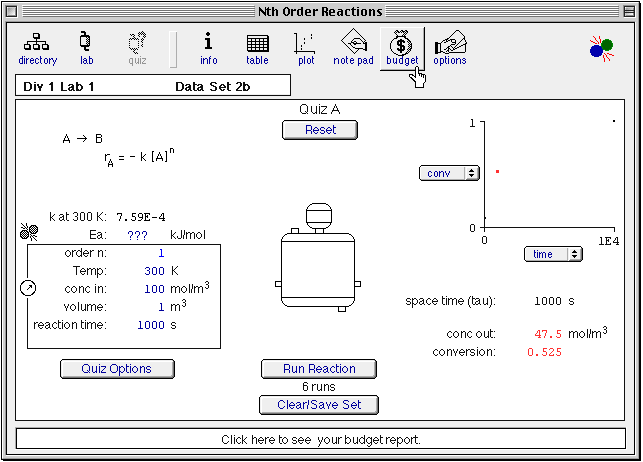Click the "info" buttons in the quiz and the lab Directory to get information on how to re-enter an unfinished quiz in a different session.Unless specifically required, don’t worry too much about whether or not you make a "profit." Do be aware that real experiments cost real time and money and that you want to design your experiments so that you can get the information you need as economically as possible.

Equations to use to get Ea and k300 from measurements at two temperatures other than 300 K.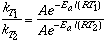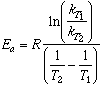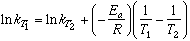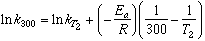Temperature, rate coefficients and activation energies in The Reactor Lab

The temperature dependence of rate coefficients is often given by the "Arrhenius equation":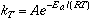where A is the "pre-exponential factor" (the value k approaches as T approaches infinity).

In The Reactor Lab, the temperature dependence is parameterized in the following way: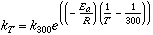where 300 K is considered the reference temperature. During analysis of experimental data, it is often preferrable to first determine a value of k with a high confidence at a reference temperature in the middle of the range of interest, and then use this equation and values of k determined at other temperatures in a regression algorithm to estimate the activation energy. This procedure minimizes parameter-correlation problems in estimates of A and Ea when fitting data with the Arrhenius equation.

With k300 chosen as an explicit input parameter, you may be able to estimate - in your head - the reaction time required to achieve a given conversion in a reactor at 300 K.

BE CAREFUL: Note that when you change values of either k300 or Ea, the value of the equivalent pre-exponential factor also changes:Home <